﻿ MechDesigner Reference & User Interface > 2.2 Mechanism-Editor: > Force elements > Add Spring FB

Use a Spring FB to add a force between two Points that are in two different Parts.

The Forces a Spring FB can exert include:

Spring Force - a force proportional to the difference of the distance between the Spring's Anchor-Point and its Free-Length.

Constant Force - a constant force that only pulls or only pushes the Spring's Anchor-Points, throughout the machine-cycle.

Coulomb Constant-Force - a constant force that acts in the opposite direction to the relative velocity between the Spring's Anchor-Point.

Drag-Force - a force that is proportional to the relative velocity between the Spring's Anchor-Points.

Drag-Force-Squared - a force that is proportional to the square of the relative velocity between the Spring's Anchor-Points.

See Spring FB dialog-box to edit these parameters.

Terminology:

 Anchor-Points : The two(2) Points that define an axis through which the Force FB applies its Force. Force element : A Spring FB is a Force-Element. Linear Motive Force : The Spring FB can become the Power-Source. It will indicate the Equivalent Linear Force that is needed to drive a Kinematic-Chain. A Linear Motor would need to provide this Force.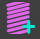1.Click Forces toolbar > Add Spring FB 2.Click two different Points - they will be the Anchor-Points -in two different Parts.

The Spring FB and its symbol are now in the graphic-area.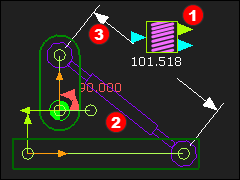Spring FB : symbols in graphic-area.

 SymbolFB - see also Spring FB connectors Symbol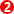Spring between the anchor-points Symbol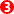Dimension between the anchor-points

Edit the Spring FB to open the Spring FB dialog-box

Note:

Positive (Negative) value will Pull (Push) the Anchor Points together (apart).

Spring FB ConnectorsOutput-Connectors

 Top : Distance, Velocity, Acceleration of the dimension between the anchor-points. Bottom : Total Force, Force-X, Force-Y acting on anchor-points.

Input-Connectors - see more at Spring FB dialog-box

 •Input a Force-Function, which we add to the Force that is defined by the Spring FB parameters. The data at the input-connector to the Spring must be Force units, (e.g. Newtons). •Convert any data-type and units with a Math FB, and use Force as the Output Data Type - see Math FB dialog-box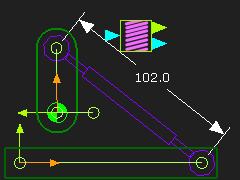The Spring FB can be in three different states.

State 1: The Spring FB is enabled.

The default state.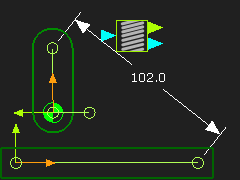State 2: The Spring FB is not enabled.

To disable the Spring FB,

1.Edit the Spring FB to open the Spring FB dialog-box

In the Spring FB dialog-box

2.Deselect the Enable Check-box.

3.Click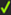to close the dialog-box.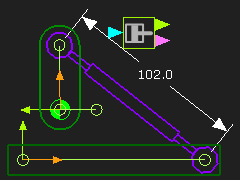State 3: The Spring FB is a Linear Motor

To change the Spring as a Linear-Motor

 1.Edit a Kinematic-Chain to open the Configure Power Source dialog-box 2.Click the Motion-Dimension FB that applies to the kinematic-chain 3.Click the Spring as the Power Source, and it becomes a Linear Motor. 4.Close the Configure Power Source dialog-box.

See how to Configure Power Source